# DeepVision: Exploiting computer vision techniques to minimize CPU Utilization¶This python notebook is for explanation of the core concepts used and the models developed for this webinar.

### Acknowledgement¶

I would like to extend my gratitude towards Open Data Science Conference, Boston team for giving me this opportunity to showcase my findings especially Alena, Vimal and Rafael.

• Software engineer working with Symantec.
• ML Researcher

### Agenda¶

• Introduction
• Tania's Story
• MNIST
• Autopilot
• Malaria Detection

### Tania's Story¶

In :
from IPython.display import YouTubeVideo

Out:

## MNIST Digit Recognition¶

In :
%%HTML

In :
from keras import Sequential
from keras.callbacks import ModelCheckpoint
from keras.datasets import mnist
import numpy as np
import matplotlib.pyplot as plt
from keras.layers import Flatten, Dense, Dropout
from keras.utils import np_utils, print_summary

Using TensorFlow backend.

In :
(x_train, y_train), (x_test, y_test) = mnist.load_data()

In :
def showData(x, label):
pixels = np.array(x, dtype='uint8')

pixels = pixels.reshape((28, 28))

plt.title('Label is {label}'.format(label=label))
plt.imshow(pixels, cmap='gray')
plt.show()

In :
showData(x_train, y_train)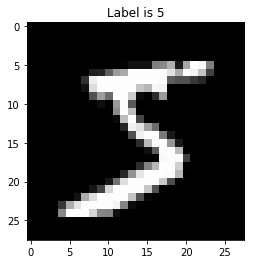In :
showData(x_train, y_train)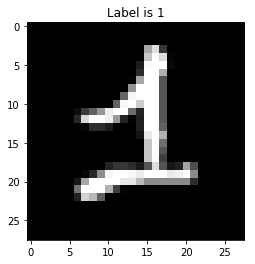In :
print(x_train.shape)

(28, 28)

In :
print(x_train)

[[  0   0   0   0   0   0   0   0   0   0   0   0   0   0   0   0   0   0
0   0   0   0   0   0   0   0   0   0]
[  0   0   0   0   0   0   0   0   0   0   0   0   0   0   0   0   0   0
0   0   0   0   0   0   0   0   0   0]
[  0   0   0   0   0   0   0   0   0   0   0   0   0   0   0   0   0   0
0   0   0   0   0   0   0   0   0   0]
[  0   0   0   0   0   0   0   0   0   0   0   0   0   0   0   0   0   0
0   0   0   0   0   0   0   0   0   0]
[  0   0   0   0   0   0   0   0   0   0   0   0   0   0   0   0   0   0
0   0   0   0   0   0   0   0   0   0]
[  0   0   0   0   0   0   0   0   0   0   0   0   3  18  18  18 126 136
175  26 166 255 247 127   0   0   0   0]
[  0   0   0   0   0   0   0   0  30  36  94 154 170 253 253 253 253 253
225 172 253 242 195  64   0   0   0   0]
[  0   0   0   0   0   0   0  49 238 253 253 253 253 253 253 253 253 251
93  82  82  56  39   0   0   0   0   0]
[  0   0   0   0   0   0   0  18 219 253 253 253 253 253 198 182 247 241
0   0   0   0   0   0   0   0   0   0]
[  0   0   0   0   0   0   0   0  80 156 107 253 253 205  11   0  43 154
0   0   0   0   0   0   0   0   0   0]
[  0   0   0   0   0   0   0   0   0  14   1 154 253  90   0   0   0   0
0   0   0   0   0   0   0   0   0   0]
[  0   0   0   0   0   0   0   0   0   0   0 139 253 190   2   0   0   0
0   0   0   0   0   0   0   0   0   0]
[  0   0   0   0   0   0   0   0   0   0   0  11 190 253  70   0   0   0
0   0   0   0   0   0   0   0   0   0]
[  0   0   0   0   0   0   0   0   0   0   0   0  35 241 225 160 108   1
0   0   0   0   0   0   0   0   0   0]
[  0   0   0   0   0   0   0   0   0   0   0   0   0  81 240 253 253 119
25   0   0   0   0   0   0   0   0   0]
[  0   0   0   0   0   0   0   0   0   0   0   0   0   0  45 186 253 253
150  27   0   0   0   0   0   0   0   0]
[  0   0   0   0   0   0   0   0   0   0   0   0   0   0   0  16  93 252
253 187   0   0   0   0   0   0   0   0]
[  0   0   0   0   0   0   0   0   0   0   0   0   0   0   0   0   0 249
253 249  64   0   0   0   0   0   0   0]
[  0   0   0   0   0   0   0   0   0   0   0   0   0   0  46 130 183 253
253 207   2   0   0   0   0   0   0   0]
[  0   0   0   0   0   0   0   0   0   0   0   0  39 148 229 253 253 253
250 182   0   0   0   0   0   0   0   0]
[  0   0   0   0   0   0   0   0   0   0  24 114 221 253 253 253 253 201
78   0   0   0   0   0   0   0   0   0]
[  0   0   0   0   0   0   0   0  23  66 213 253 253 253 253 198  81   2
0   0   0   0   0   0   0   0   0   0]
[  0   0   0   0   0   0  18 171 219 253 253 253 253 195  80   9   0   0
0   0   0   0   0   0   0   0   0   0]
[  0   0   0   0  55 172 226 253 253 253 253 244 133  11   0   0   0   0
0   0   0   0   0   0   0   0   0   0]
[  0   0   0   0 136 253 253 253 212 135 132  16   0   0   0   0   0   0
0   0   0   0   0   0   0   0   0   0]
[  0   0   0   0   0   0   0   0   0   0   0   0   0   0   0   0   0   0
0   0   0   0   0   0   0   0   0   0]
[  0   0   0   0   0   0   0   0   0   0   0   0   0   0   0   0   0   0
0   0   0   0   0   0   0   0   0   0]
[  0   0   0   0   0   0   0   0   0   0   0   0   0   0   0   0   0   0
0   0   0   0   0   0   0   0   0   0]]


### Normalization¶

Normalization is a technique often applied as part of data preparation for machine learning. The goal of normalization is to change the values of numeric columns in the dataset to a common scale, without distorting differences in the ranges of values

In :
x_train_norm= x_train / 255.
x_test_norm=x_test / 255.

In :
print(x_train_norm.shape)

(28, 28)

In :
print(x_train_norm)

[[0.         0.         0.         0.         0.         0.
0.         0.         0.         0.         0.         0.
0.         0.         0.         0.         0.         0.
0.         0.         0.         0.         0.         0.
0.         0.         0.         0.        ]
[0.         0.         0.         0.         0.         0.
0.         0.         0.         0.         0.         0.
0.         0.         0.         0.         0.         0.
0.         0.         0.         0.         0.         0.
0.         0.         0.         0.        ]
[0.         0.         0.         0.         0.         0.
0.         0.         0.         0.         0.         0.
0.         0.         0.         0.         0.         0.
0.         0.         0.         0.         0.         0.
0.         0.         0.         0.        ]
[0.         0.         0.         0.         0.         0.
0.         0.         0.         0.         0.         0.
0.         0.         0.         0.         0.         0.
0.         0.         0.         0.         0.         0.
0.         0.         0.         0.        ]
[0.         0.         0.         0.         0.         0.
0.         0.         0.         0.         0.         0.
0.         0.         0.         0.         0.         0.
0.         0.         0.         0.         0.         0.
0.         0.         0.         0.        ]
[0.         0.         0.         0.         0.         0.
0.         0.         0.         0.         0.         0.
0.01176471 0.07058824 0.07058824 0.07058824 0.49411765 0.53333333
0.68627451 0.10196078 0.65098039 1.         0.96862745 0.49803922
0.         0.         0.         0.        ]
[0.         0.         0.         0.         0.         0.
0.         0.         0.11764706 0.14117647 0.36862745 0.60392157
0.66666667 0.99215686 0.99215686 0.99215686 0.99215686 0.99215686
0.88235294 0.6745098  0.99215686 0.94901961 0.76470588 0.25098039
0.         0.         0.         0.        ]
[0.         0.         0.         0.         0.         0.
0.         0.19215686 0.93333333 0.99215686 0.99215686 0.99215686
0.99215686 0.99215686 0.99215686 0.99215686 0.99215686 0.98431373
0.36470588 0.32156863 0.32156863 0.21960784 0.15294118 0.
0.         0.         0.         0.        ]
[0.         0.         0.         0.         0.         0.
0.         0.07058824 0.85882353 0.99215686 0.99215686 0.99215686
0.99215686 0.99215686 0.77647059 0.71372549 0.96862745 0.94509804
0.         0.         0.         0.         0.         0.
0.         0.         0.         0.        ]
[0.         0.         0.         0.         0.         0.
0.         0.         0.31372549 0.61176471 0.41960784 0.99215686
0.99215686 0.80392157 0.04313725 0.         0.16862745 0.60392157
0.         0.         0.         0.         0.         0.
0.         0.         0.         0.        ]
[0.         0.         0.         0.         0.         0.
0.         0.         0.         0.05490196 0.00392157 0.60392157
0.99215686 0.35294118 0.         0.         0.         0.
0.         0.         0.         0.         0.         0.
0.         0.         0.         0.        ]
[0.         0.         0.         0.         0.         0.
0.         0.         0.         0.         0.         0.54509804
0.99215686 0.74509804 0.00784314 0.         0.         0.
0.         0.         0.         0.         0.         0.
0.         0.         0.         0.        ]
[0.         0.         0.         0.         0.         0.
0.         0.         0.         0.         0.         0.04313725
0.74509804 0.99215686 0.2745098  0.         0.         0.
0.         0.         0.         0.         0.         0.
0.         0.         0.         0.        ]
[0.         0.         0.         0.         0.         0.
0.         0.         0.         0.         0.         0.
0.1372549  0.94509804 0.88235294 0.62745098 0.42352941 0.00392157
0.         0.         0.         0.         0.         0.
0.         0.         0.         0.        ]
[0.         0.         0.         0.         0.         0.
0.         0.         0.         0.         0.         0.
0.         0.31764706 0.94117647 0.99215686 0.99215686 0.46666667
0.09803922 0.         0.         0.         0.         0.
0.         0.         0.         0.        ]
[0.         0.         0.         0.         0.         0.
0.         0.         0.         0.         0.         0.
0.         0.         0.17647059 0.72941176 0.99215686 0.99215686
0.58823529 0.10588235 0.         0.         0.         0.
0.         0.         0.         0.        ]
[0.         0.         0.         0.         0.         0.
0.         0.         0.         0.         0.         0.
0.         0.         0.         0.0627451  0.36470588 0.98823529
0.99215686 0.73333333 0.         0.         0.         0.
0.         0.         0.         0.        ]
[0.         0.         0.         0.         0.         0.
0.         0.         0.         0.         0.         0.
0.         0.         0.         0.         0.         0.97647059
0.99215686 0.97647059 0.25098039 0.         0.         0.
0.         0.         0.         0.        ]
[0.         0.         0.         0.         0.         0.
0.         0.         0.         0.         0.         0.
0.         0.         0.18039216 0.50980392 0.71764706 0.99215686
0.99215686 0.81176471 0.00784314 0.         0.         0.
0.         0.         0.         0.        ]
[0.         0.         0.         0.         0.         0.
0.         0.         0.         0.         0.         0.
0.15294118 0.58039216 0.89803922 0.99215686 0.99215686 0.99215686
0.98039216 0.71372549 0.         0.         0.         0.
0.         0.         0.         0.        ]
[0.         0.         0.         0.         0.         0.
0.         0.         0.         0.         0.09411765 0.44705882
0.86666667 0.99215686 0.99215686 0.99215686 0.99215686 0.78823529
0.30588235 0.         0.         0.         0.         0.
0.         0.         0.         0.        ]
[0.         0.         0.         0.         0.         0.
0.         0.         0.09019608 0.25882353 0.83529412 0.99215686
0.99215686 0.99215686 0.99215686 0.77647059 0.31764706 0.00784314
0.         0.         0.         0.         0.         0.
0.         0.         0.         0.        ]
[0.         0.         0.         0.         0.         0.
0.07058824 0.67058824 0.85882353 0.99215686 0.99215686 0.99215686
0.99215686 0.76470588 0.31372549 0.03529412 0.         0.
0.         0.         0.         0.         0.         0.
0.         0.         0.         0.        ]
[0.         0.         0.         0.         0.21568627 0.6745098
0.88627451 0.99215686 0.99215686 0.99215686 0.99215686 0.95686275
0.52156863 0.04313725 0.         0.         0.         0.
0.         0.         0.         0.         0.         0.
0.         0.         0.         0.        ]
[0.         0.         0.         0.         0.53333333 0.99215686
0.99215686 0.99215686 0.83137255 0.52941176 0.51764706 0.0627451
0.         0.         0.         0.         0.         0.
0.         0.         0.         0.         0.         0.
0.         0.         0.         0.        ]
[0.         0.         0.         0.         0.         0.
0.         0.         0.         0.         0.         0.
0.         0.         0.         0.         0.         0.
0.         0.         0.         0.         0.         0.
0.         0.         0.         0.        ]
[0.         0.         0.         0.         0.         0.
0.         0.         0.         0.         0.         0.
0.         0.         0.         0.         0.         0.
0.         0.         0.         0.         0.         0.
0.         0.         0.         0.        ]
[0.         0.         0.         0.         0.         0.
0.         0.         0.         0.         0.         0.
0.         0.         0.         0.         0.         0.
0.         0.         0.         0.         0.         0.
0.         0.         0.         0.        ]]

In :
x_train_norm_mean_zero= x_train / 127.5 - 1.
x_test_norm_mean_zero=x_test / 127.5 - 1.

In :
print(x_train_norm_mean_zero.shape)

(28, 28)

In :
print(x_train_norm_mean_zero)

[[-1.         -1.         -1.         -1.         -1.         -1.
-1.         -1.         -1.         -1.         -1.         -1.
-1.         -1.         -1.         -1.         -1.         -1.
-1.         -1.         -1.         -1.         -1.         -1.
-1.         -1.         -1.         -1.        ]
[-1.         -1.         -1.         -1.         -1.         -1.
-1.         -1.         -1.         -1.         -1.         -1.
-1.         -1.         -1.         -1.         -1.         -1.
-1.         -1.         -1.         -1.         -1.         -1.
-1.         -1.         -1.         -1.        ]
[-1.         -1.         -1.         -1.         -1.         -1.
-1.         -1.         -1.         -1.         -1.         -1.
-1.         -1.         -1.         -1.         -1.         -1.
-1.         -1.         -1.         -1.         -1.         -1.
-1.         -1.         -1.         -1.        ]
[-1.         -1.         -1.         -1.         -1.         -1.
-1.         -1.         -1.         -1.         -1.         -1.
-1.         -1.         -1.         -1.         -1.         -1.
-1.         -1.         -1.         -1.         -1.         -1.
-1.         -1.         -1.         -1.        ]
[-1.         -1.         -1.         -1.         -1.         -1.
-1.         -1.         -1.         -1.         -1.         -1.
-1.         -1.         -1.         -1.         -1.         -1.
-1.         -1.         -1.         -1.         -1.         -1.
-1.         -1.         -1.         -1.        ]
[-1.         -1.         -1.         -1.         -1.         -1.
-1.         -1.         -1.         -1.         -1.         -1.
-0.97647059 -0.85882353 -0.85882353 -0.85882353 -0.01176471  0.06666667
0.37254902 -0.79607843  0.30196078  1.          0.9372549  -0.00392157
-1.         -1.         -1.         -1.        ]
[-1.         -1.         -1.         -1.         -1.         -1.
-1.         -1.         -0.76470588 -0.71764706 -0.2627451   0.20784314
0.33333333  0.98431373  0.98431373  0.98431373  0.98431373  0.98431373
0.76470588  0.34901961  0.98431373  0.89803922  0.52941176 -0.49803922
-1.         -1.         -1.         -1.        ]
[-1.         -1.         -1.         -1.         -1.         -1.
-1.         -0.61568627  0.86666667  0.98431373  0.98431373  0.98431373
0.98431373  0.98431373  0.98431373  0.98431373  0.98431373  0.96862745
-0.27058824 -0.35686275 -0.35686275 -0.56078431 -0.69411765 -1.
-1.         -1.         -1.         -1.        ]
[-1.         -1.         -1.         -1.         -1.         -1.
-1.         -0.85882353  0.71764706  0.98431373  0.98431373  0.98431373
0.98431373  0.98431373  0.55294118  0.42745098  0.9372549   0.89019608
-1.         -1.         -1.         -1.         -1.         -1.
-1.         -1.         -1.         -1.        ]
[-1.         -1.         -1.         -1.         -1.         -1.
-1.         -1.         -0.37254902  0.22352941 -0.16078431  0.98431373
0.98431373  0.60784314 -0.91372549 -1.         -0.6627451   0.20784314
-1.         -1.         -1.         -1.         -1.         -1.
-1.         -1.         -1.         -1.        ]
[-1.         -1.         -1.         -1.         -1.         -1.
-1.         -1.         -1.         -0.89019608 -0.99215686  0.20784314
0.98431373 -0.29411765 -1.         -1.         -1.         -1.
-1.         -1.         -1.         -1.         -1.         -1.
-1.         -1.         -1.         -1.        ]
[-1.         -1.         -1.         -1.         -1.         -1.
-1.         -1.         -1.         -1.         -1.          0.09019608
0.98431373  0.49019608 -0.98431373 -1.         -1.         -1.
-1.         -1.         -1.         -1.         -1.         -1.
-1.         -1.         -1.         -1.        ]
[-1.         -1.         -1.         -1.         -1.         -1.
-1.         -1.         -1.         -1.         -1.         -0.91372549
0.49019608  0.98431373 -0.45098039 -1.         -1.         -1.
-1.         -1.         -1.         -1.         -1.         -1.
-1.         -1.         -1.         -1.        ]
[-1.         -1.         -1.         -1.         -1.         -1.
-1.         -1.         -1.         -1.         -1.         -1.
-0.7254902   0.89019608  0.76470588  0.25490196 -0.15294118 -0.99215686
-1.         -1.         -1.         -1.         -1.         -1.
-1.         -1.         -1.         -1.        ]
[-1.         -1.         -1.         -1.         -1.         -1.
-1.         -1.         -1.         -1.         -1.         -1.
-1.         -0.36470588  0.88235294  0.98431373  0.98431373 -0.06666667
-0.80392157 -1.         -1.         -1.         -1.         -1.
-1.         -1.         -1.         -1.        ]
[-1.         -1.         -1.         -1.         -1.         -1.
-1.         -1.         -1.         -1.         -1.         -1.
-1.         -1.         -0.64705882  0.45882353  0.98431373  0.98431373
0.17647059 -0.78823529 -1.         -1.         -1.         -1.
-1.         -1.         -1.         -1.        ]
[-1.         -1.         -1.         -1.         -1.         -1.
-1.         -1.         -1.         -1.         -1.         -1.
-1.         -1.         -1.         -0.8745098  -0.27058824  0.97647059
0.98431373  0.46666667 -1.         -1.         -1.         -1.
-1.         -1.         -1.         -1.        ]
[-1.         -1.         -1.         -1.         -1.         -1.
-1.         -1.         -1.         -1.         -1.         -1.
-1.         -1.         -1.         -1.         -1.          0.95294118
0.98431373  0.95294118 -0.49803922 -1.         -1.         -1.
-1.         -1.         -1.         -1.        ]
[-1.         -1.         -1.         -1.         -1.         -1.
-1.         -1.         -1.         -1.         -1.         -1.
-1.         -1.         -0.63921569  0.01960784  0.43529412  0.98431373
0.98431373  0.62352941 -0.98431373 -1.         -1.         -1.
-1.         -1.         -1.         -1.        ]
[-1.         -1.         -1.         -1.         -1.         -1.
-1.         -1.         -1.         -1.         -1.         -1.
-0.69411765  0.16078431  0.79607843  0.98431373  0.98431373  0.98431373
0.96078431  0.42745098 -1.         -1.         -1.         -1.
-1.         -1.         -1.         -1.        ]
[-1.         -1.         -1.         -1.         -1.         -1.
-1.         -1.         -1.         -1.         -0.81176471 -0.10588235
0.73333333  0.98431373  0.98431373  0.98431373  0.98431373  0.57647059
-0.38823529 -1.         -1.         -1.         -1.         -1.
-1.         -1.         -1.         -1.        ]
[-1.         -1.         -1.         -1.         -1.         -1.
-1.         -1.         -0.81960784 -0.48235294  0.67058824  0.98431373
0.98431373  0.98431373  0.98431373  0.55294118 -0.36470588 -0.98431373
-1.         -1.         -1.         -1.         -1.         -1.
-1.         -1.         -1.         -1.        ]
[-1.         -1.         -1.         -1.         -1.         -1.
-0.85882353  0.34117647  0.71764706  0.98431373  0.98431373  0.98431373
0.98431373  0.52941176 -0.37254902 -0.92941176 -1.         -1.
-1.         -1.         -1.         -1.         -1.         -1.
-1.         -1.         -1.         -1.        ]
[-1.         -1.         -1.         -1.         -0.56862745  0.34901961
0.77254902  0.98431373  0.98431373  0.98431373  0.98431373  0.91372549
0.04313725 -0.91372549 -1.         -1.         -1.         -1.
-1.         -1.         -1.         -1.         -1.         -1.
-1.         -1.         -1.         -1.        ]
[-1.         -1.         -1.         -1.          0.06666667  0.98431373
0.98431373  0.98431373  0.6627451   0.05882353  0.03529412 -0.8745098
-1.         -1.         -1.         -1.         -1.         -1.
-1.         -1.         -1.         -1.         -1.         -1.
-1.         -1.         -1.         -1.        ]
[-1.         -1.         -1.         -1.         -1.         -1.
-1.         -1.         -1.         -1.         -1.         -1.
-1.         -1.         -1.         -1.         -1.         -1.
-1.         -1.         -1.         -1.         -1.         -1.
-1.         -1.         -1.         -1.        ]
[-1.         -1.         -1.         -1.         -1.         -1.
-1.         -1.         -1.         -1.         -1.         -1.
-1.         -1.         -1.         -1.         -1.         -1.
-1.         -1.         -1.         -1.         -1.         -1.
-1.         -1.         -1.         -1.        ]
[-1.         -1.         -1.         -1.         -1.         -1.
-1.         -1.         -1.         -1.         -1.         -1.
-1.         -1.         -1.         -1.         -1.         -1.
-1.         -1.         -1.         -1.         -1.         -1.
-1.         -1.         -1.         -1.        ]]

In :
def preprocess_labels(y):
labels = np_utils.to_categorical(y)
return labels

In :
y_train = preprocess_labels(y_train)
y_test = preprocess_labels(y_test)

In :
x_train = x_train.reshape(x_train.shape, 28, 28, 1)
x_test = x_test.reshape(x_test.shape, 28, 28, 1)

x_train_norm = x_train_norm.reshape(x_train_norm.shape, 28, 28, 1)
x_test_norm = x_test_norm.reshape(x_test_norm.shape, 28, 28, 1)

x_train_norm_mean_zero = x_train_norm_mean_zero.reshape(x_train_norm_mean_zero.shape, 28, 28, 1)
x_test_norm_mean_zero = x_test_norm_mean_zero.reshape(x_test_norm_mean_zero.shape, 28, 28, 1)

In :
print("number of training examples = " + str(x_train.shape))
print("number of test examples = " + str(x_test.shape))
print("X_train shape: " + str(x_train.shape))
print("Y_train shape: " + str(y_train.shape))

number of training examples = 60000
number of test examples = 10000
X_train shape: (60000, 28, 28, 1)
Y_train shape: (60000, 10)

In :
def keras_model(image_x, image_y):
num_of_classes = 10
model = Sequential()

filepath = "mnist_odsc.h5"
checkpoint = ModelCheckpoint(filepath, monitor='val_acc', verbose=1, save_best_only=True, mode='max')
callbacks_list = [checkpoint]

return model, callbacks_list

model, callbacks_list = keras_model(28, 28)
print_summary(model)

_________________________________________________________________
Layer (type)                 Output Shape              Param #
=================================================================
flatten_3 (Flatten)          (None, 784)               0
_________________________________________________________________
dense_7 (Dense)              (None, 512)               401920
_________________________________________________________________
dropout_5 (Dropout)          (None, 512)               0
_________________________________________________________________
dense_8 (Dense)              (None, 128)               65664
_________________________________________________________________
dropout_6 (Dropout)          (None, 128)               0
_________________________________________________________________
dense_9 (Dense)              (None, 10)                1290
=================================================================
Total params: 468,874
Trainable params: 468,874
Non-trainable params: 0
_________________________________________________________________

In :
model.fit(x_train, y_train, validation_data=(x_test, y_test), epochs=1, batch_size=64,
callbacks=callbacks_list)

Train on 60000 samples, validate on 10000 samples
Epoch 1/1
60000/60000 [==============================] - 22s 371us/step - loss: 12.5177 - acc: 0.2218 - val_loss: 11.3287 - val_acc: 0.2968

Epoch 00001: val_acc improved from -inf to 0.29680, saving model to mnist_odsc.h5

Out:
<keras.callbacks.History at 0x25183d5a860>
In :
model.fit(x_train_norm, y_train, validation_data=(x_test_norm, y_test), epochs=1, batch_size=64,
callbacks=callbacks_list)

Train on 60000 samples, validate on 10000 samples
Epoch 1/1
60000/60000 [==============================] - 19s 323us/step - loss: 1.1754 - acc: 0.6667 - val_loss: 0.3117 - val_acc: 0.9128

Epoch 00001: val_acc improved from 0.29680 to 0.91280, saving model to mnist_odsc.h5

Out:
<keras.callbacks.History at 0x25183d5a9e8>
In :
model.fit(x_train_norm_mean_zero, y_train, validation_data=(x_test_norm_mean_zero, y_test), epochs=1, batch_size=64,
callbacks=callbacks_list)

Train on 60000 samples, validate on 10000 samples
Epoch 1/1
60000/60000 [==============================] - 20s 341us/step - loss: 0.9066 - acc: 0.7362 - val_loss: 0.3179 - val_acc: 0.9155

Epoch 00001: val_acc improved from 0.91280 to 0.91550, saving model to mnist_odsc.h5

Out:
<keras.callbacks.History at 0x25186ab3e10>

## Autopilot¶

This code helps in getting the steering angle of self driving car. The inspiraion is taken from Udacity Self driving car module as well End to End Learning for Self-Driving Cars module from NVIDIA

The End to End Learning for Self-Driving Cars research paper can be found at (https://arxiv.org/abs/1604.07316) This repository uses convnets to predict steering angle according to the road.

1) Autopilot Version 1 2) Autopilot Version 2

### Code Requirements¶

You can install Conda for python which resolves all the dependencies for machine learning.

### Description¶

An autonomous car (also known as a driverless car, self-driving car, and robotic car) is a vehicle that is capable of sensing its environment and navigating without human input. Autonomous cars combine a variety of techniques to perceive their surroundings, including radar, laser light, GPS, odometry, and computer vision. Advanced control systems interpret sensory information to identify appropriate navigation paths, as well as obstacles and relevant signage

## Autopilot V1 (Udacity Dataset based on Udacity Simulator)¶

### Dataset¶

You can get the dataset at here

## Autopilot V2 (NVIDIA Dataset based on real world)¶

### References:¶

In :
%%HTML

In :
from __future__ import division
import cv2
import os
import numpy as np
import scipy
import pickle
import matplotlib.pyplot as plt
from itertools import islice

In :
DATA_FOLDER = 'driving_dataset'
TRAIN_FILE = os.path.join(DATA_FOLDER, 'data.txt')

In :
def showData(x, label):
pixels = np.array(img, dtype='uint8')

pixels = pixels.reshape((256, 455,3))

plt.title('Label is {label}'.format(label=label))
plt.imshow(pixels, cmap='gray')
plt.show()

In :
showData("F:\\projects\\SIT_Sample\\AutoPilot\\driving_dataset\\500.jpg",1)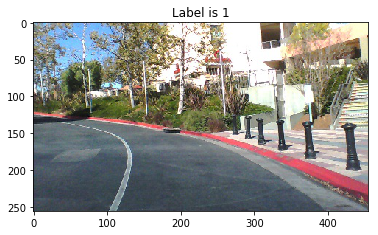In :
showData("F:\\projects\\SIT_Sample\\AutoPilot\\driving_dataset\\595.jpg",1)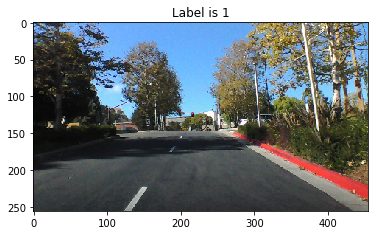In :
def preprocess(img):
resized = cv2.resize((cv2.cvtColor(img, cv2.COLOR_RGB2HSV))[:, :, 1], (100, 100))
return resized

In :
def showData_HSV(x, label):
img=preprocess(img)
pixels = np.array(img, dtype='uint8')

pixels = pixels.reshape((100, 100))

plt.title('Label is {label}'.format(label=label))
plt.imshow(pixels, cmap='gray')
plt.show()

In :
showData_HSV("F:\\projects\\SIT_Sample\\AutoPilot\\driving_dataset\\500.jpg",1)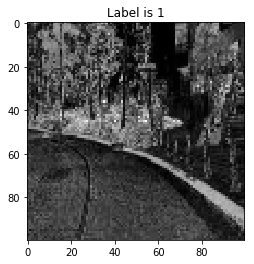In :
showData_HSV("F:\\projects\\SIT_Sample\\AutoPilot\\driving_dataset\\595.jpg",1)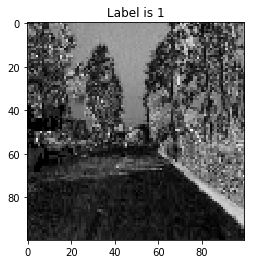In :
#Build the model
import numpy as np
from keras.layers import Dense, Activation, Flatten, Conv2D, Lambda
from keras.layers import MaxPooling2D, Dropout
from keras.utils import print_summary
from keras.models import Sequential
from keras.callbacks import ModelCheckpoint
import keras.backend as K
import pickle

from sklearn.model_selection import train_test_split
from sklearn.utils import shuffle

In :
# This excerpt of code collects images and the steering angle, does pre processing and stores in a pickle file
def return_data():

X = []
y = []
features = []

with open(TRAIN_FILE) as fp:
for line in islice(fp, LIMIT):
path, angle = line.strip().split()
full_path = os.path.join(DATA_FOLDER, path)
X.append(full_path)
# using angles from -pi to pi to avoid rescaling the atan in the network
y.append(float(angle) * scipy.pi / 180)

for i in range(len(X)):
features.append(preprocess(img))

features = np.array(features).astype('float32')
labels = np.array(y).astype('float32')

with open("features", "wb") as f:
pickle.dump(features, f, protocol=4)
with open("labels", "wb") as f:
pickle.dump(labels, f, protocol=4)

In :
def loadFromPickle():
with open("features", "rb") as f:
with open("labels", "rb") as f:

return features, labels

In :
features, labels = loadFromPickle()

In :
features, labels = shuffle(features, labels)
train_x, test_x, train_y, test_y = train_test_split(features, labels, random_state=0,
test_size=0.3)
train_x = train_x.reshape(train_x.shape, 100, 100, 1)
test_x = test_x.reshape(test_x.shape, 100, 100, 1)

In :
print("number of training examples = " + str(train_x.shape))
print("number of test examples = " + str(test_x.shape))
print("X_train shape: " + str(train_x.shape))

number of training examples = 31784
number of test examples = 13622
X_train shape: (31784, 100, 100, 1)

In :
def showLoadedData(x, label):
pixels = np.array(x, dtype='uint8')

#pixels = pixels.reshape((100, 100))

plt.title('Label is {label}'.format(label=label))
plt.imshow(pixels, cmap='gray')
plt.show()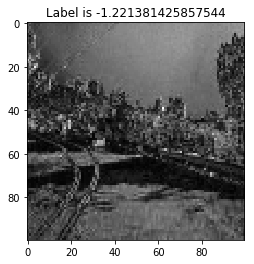In :
from keras.layers import BatchNormalization,Input
from keras.layers.convolutional import Convolution2D
import tensorflow as tf
from keras.models import Model

def atan(x):
return tf.atan(x)

In :
#Lets look at the model for the original research paper

def paper_model():
inputs = Input(shape=(66, 200, 3))
conv_1 = Convolution2D(24, 5, 5, activation='relu', name='conv_1', subsample=(2, 2))(inputs)
conv_2 = Convolution2D(36, 5, 5, activation='relu', name='conv_2', subsample=(2, 2))(conv_1)
conv_3 = Convolution2D(48, 5, 5, activation='relu', name='conv_3', subsample=(2, 2))(conv_2)
conv_3 = Dropout(.5)(conv_3)

conv_4 = Convolution2D(64, 3, 3, activation='relu', name='conv_4', subsample=(1, 1))(conv_3)
conv_5 = Convolution2D(64, 3, 3, activation='relu', name='conv_5', subsample=(1, 1))(conv_4)

flat = Flatten()(conv_5)

dense_1 = Dense(1164)(flat)
dense_1 = Dropout(.5)(flat)
dense_2 = Dense(100, activation='relu')(dense_1)
dense_2 = Dropout(.5)(flat)
dense_3 = Dense(50, activation='relu')(dense_2)
dense_3 = Dropout(.5)(flat)
dense_4 = Dense(10, activation='relu')(dense_3)
dense_4 = Dropout(.5)(flat)

final = Dense(1, activation=atan)(dense_4)
model = Model(input=inputs, output=final)

return model

In :
model=paper_model()

F:\Anaconda3\lib\site-packages\ipykernel_launcher.py:5: UserWarning: Update your Conv2D call to the Keras 2 API: Conv2D(24, (5, 5), activation="relu", name="conv_1", strides=(2, 2))
"""
F:\Anaconda3\lib\site-packages\ipykernel_launcher.py:6: UserWarning: Update your Conv2D call to the Keras 2 API: Conv2D(36, (5, 5), activation="relu", name="conv_2", strides=(2, 2))

F:\Anaconda3\lib\site-packages\ipykernel_launcher.py:7: UserWarning: Update your Conv2D call to the Keras 2 API: Conv2D(48, (5, 5), activation="relu", name="conv_3", strides=(2, 2))
import sys
F:\Anaconda3\lib\site-packages\ipykernel_launcher.py:10: UserWarning: Update your Conv2D call to the Keras 2 API: Conv2D(64, (3, 3), activation="relu", name="conv_4", strides=(1, 1))
# Remove the CWD from sys.path while we load stuff.
F:\Anaconda3\lib\site-packages\ipykernel_launcher.py:11: UserWarning: Update your Conv2D call to the Keras 2 API: Conv2D(64, (3, 3), activation="relu", name="conv_5", strides=(1, 1))
# This is added back by InteractiveShellApp.init_path()
F:\Anaconda3\lib\site-packages\ipykernel_launcher.py:25: UserWarning: Update your Model call to the Keras 2 API: Model(inputs=Tensor("in..., outputs=Tensor("de...)

In :
print_summary(model)

_________________________________________________________________
Layer (type)                 Output Shape              Param #
=================================================================
input_5 (InputLayer)         (None, 66, 200, 3)        0
_________________________________________________________________
conv_1 (Conv2D)              (None, 31, 98, 24)        1824
_________________________________________________________________
conv_2 (Conv2D)              (None, 14, 47, 36)        21636
_________________________________________________________________
conv_3 (Conv2D)              (None, 5, 22, 48)         43248
_________________________________________________________________
dropout_27 (Dropout)         (None, 5, 22, 48)         0
_________________________________________________________________
conv_4 (Conv2D)              (None, 3, 20, 64)         27712
_________________________________________________________________
conv_5 (Conv2D)              (None, 1, 18, 64)         36928
_________________________________________________________________
flatten_8 (Flatten)          (None, 1152)              0
_________________________________________________________________
dropout_31 (Dropout)         (None, 1152)              0
_________________________________________________________________
dense_33 (Dense)             (None, 1)                 1153
=================================================================
Total params: 132,501
Trainable params: 132,501
Non-trainable params: 0
_________________________________________________________________

In :
def keras_model(image_x, image_y):
model = Sequential()
model.add(Lambda(lambda x: x / 127.5 - 1., input_shape=(image_x, image_y, 1)))

filepath = "Autopilot.h5"
checkpoint = ModelCheckpoint(filepath, verbose=1, save_best_only=True)
callbacks_list = [checkpoint]

return model, callbacks_list

In :
model, callbacks_list = keras_model(100, 100)
print_summary(model)

_________________________________________________________________
Layer (type)                 Output Shape              Param #
=================================================================
lambda_13 (Lambda)           (None, 100, 100, 1)       0
_________________________________________________________________
conv2d_30 (Conv2D)           (None, 100, 100, 16)      416
_________________________________________________________________
activation_25 (Activation)   (None, 100, 100, 16)      0
_________________________________________________________________
max_pooling2d_25 (MaxPooling (None, 20, 20, 16)        0
_________________________________________________________________
conv2d_31 (Conv2D)           (None, 20, 20, 32)        12832
_________________________________________________________________
activation_26 (Activation)   (None, 20, 20, 32)        0
_________________________________________________________________
max_pooling2d_26 (MaxPooling (None, 4, 4, 32)          0
_________________________________________________________________
flatten_21 (Flatten)         (None, 512)               0
_________________________________________________________________
dropout_44 (Dropout)         (None, 512)               0
_________________________________________________________________
dense_63 (Dense)             (None, 128)               65664
_________________________________________________________________
dense_64 (Dense)             (None, 10)                1290
_________________________________________________________________
dense_65 (Dense)             (None, 1)                 11
=================================================================
Total params: 80,213
Trainable params: 80,213
Non-trainable params: 0
_________________________________________________________________

In :
model.fit(train_x, train_y, validation_data=(test_x, test_y), epochs=1, batch_size=32,
callbacks=callbacks_list)

Train on 31784 samples, validate on 13622 samples
Epoch 1/1
5152/31784 [===>..........................] - ETA: 8:42 - loss: 0.3135
---------------------------------------------------------------------------
KeyboardInterrupt                         Traceback (most recent call last)
<ipython-input-195-fb5193bdc8bf> in <module>()
1 model.fit(train_x, train_y, validation_data=(test_x, test_y), epochs=1, batch_size=32,
----> 2               callbacks=callbacks_list)

F:\Anaconda3\lib\site-packages\keras\engine\training.py in fit(self, x, y, batch_size, epochs, verbose, callbacks, validation_split, validation_data, shuffle, class_weight, sample_weight, initial_epoch, steps_per_epoch, validation_steps, **kwargs)
1037                                         initial_epoch=initial_epoch,
1038                                         steps_per_epoch=steps_per_epoch,
-> 1039                                         validation_steps=validation_steps)
1040
1041     def evaluate(self, x=None, y=None,

F:\Anaconda3\lib\site-packages\keras\engine\training_arrays.py in fit_loop(model, f, ins, out_labels, batch_size, epochs, verbose, callbacks, val_f, val_ins, shuffle, callback_metrics, initial_epoch, steps_per_epoch, validation_steps)
197                     ins_batch[i] = ins_batch[i].toarray()
198
--> 199                 outs = f(ins_batch)
200                 outs = to_list(outs)
201                 for l, o in zip(out_labels, outs):

F:\Anaconda3\lib\site-packages\keras\backend\tensorflow_backend.py in __call__(self, inputs)
2713                 return self._legacy_call(inputs)
2714
-> 2715             return self._call(inputs)
2716         else:
2717             if py_any(is_tensor(x) for x in inputs):

F:\Anaconda3\lib\site-packages\keras\backend\tensorflow_backend.py in _call(self, inputs)
2674         else:
-> 2675             fetched = self._callable_fn(*array_vals)
2676         return fetched[:len(self.outputs)]
2677

F:\Anaconda3\lib\site-packages\tensorflow\python\client\session.py in __call__(self, *args, **kwargs)
1437           ret = tf_session.TF_SessionRunCallable(
1438               self._session._session, self._handle, args, status,

KeyboardInterrupt: 

## Malaria Detection¶

In :
import matplotlib.pyplot as plt
from PIL import Image
import numpy as np
from matplotlib.pyplot import imshow
import cv2
image = Image.open(x)
plt.imshow(np.asarray(image), cmap='gray')
print(image.size)
plt.show()


(142, 163)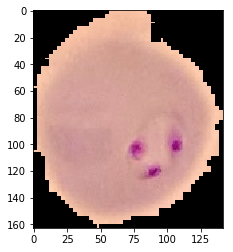In :
showMalariaData("F:\\projects\\\Malaria_Detection\\cell_images\\Parasitized\\C39P4thinF_original_IMG_20150622_105335_cell_6.png")

(100, 94)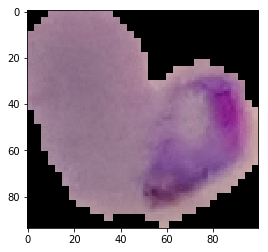In :
# Let's look at normal cells

(127, 136)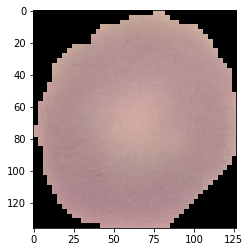In :
showMalariaData("F:\\projects\\\Malaria_Detection\\cell_images\\Uninfected\\C122P83ThinF_IMG_20151002_145014_cell_158.png")

(121, 148)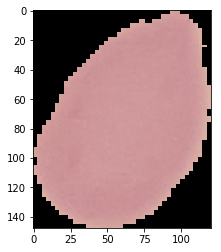In :
# Let's add some filters and see if we can remove some noise
def showMalariaFiltered_HLS_Data(x):
image = Image.open(x)
image=np.asarray(image)
hsv = cv2.cvtColor(image, cv2.COLOR_RGB2HLS)
plt.imshow(hsv, cmap='gray')
print(hsv.size)
plt.show()

showMalariaFiltered_HLS_Data("F:\\projects\\\Malaria_Detection\\cell_images\\Parasitized\\C33P1thinF_IMG_20150619_114756a_cell_179.png")

69438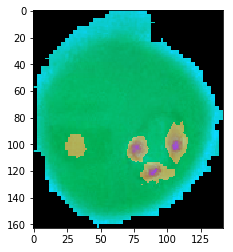In :
showMalariaFiltered_HLS_Data("F:\\projects\\\Malaria_Detection\\cell_images\\Uninfected\\C1_thinF_IMG_20150604_104722_cell_60.png")

51816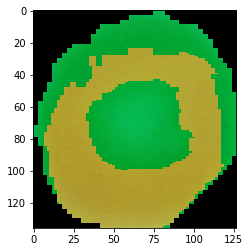In :
def showMalariaFiltered_HSV_Data(x):
image = Image.open(x)
image=np.asarray(image)
hsv = cv2.cvtColor(image, cv2.COLOR_RGB2HSV)
plt.imshow(hsv, cmap='gray')
print(hsv.size)
plt.show()

In :
showMalariaFiltered_HSV_Data("F:\\projects\\\Malaria_Detection\\cell_images\\Parasitized\\C33P1thinF_IMG_20150619_114756a_cell_179.png")

69438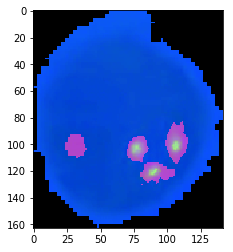In :
showMalariaFiltered_HSV_Data("F:\\projects\\\Malaria_Detection\\cell_images\\Uninfected\\C1_thinF_IMG_20150604_104722_cell_60.png")

51816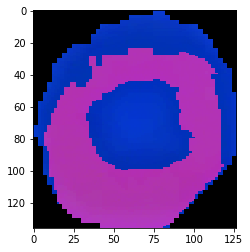In :
def showMalariaFiltered_LAB_Data(x):
image = Image.open(x)
image=np.asarray(image)
hsv = cv2.cvtColor(image, cv2.COLOR_BGR2LAB)
plt.imshow(hsv, cmap='gray')
print(hsv.size)
plt.show()

In :
showMalariaFiltered_LAB_Data("F:\\projects\\\Malaria_Detection\\cell_images\\Parasitized\\C33P1thinF_IMG_20150619_114756a_cell_179.png")

69438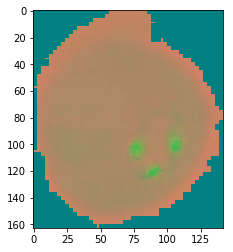In :
showMalariaFiltered_LAB_Data("F:\\projects\\\Malaria_Detection\\cell_images\\Uninfected\\C1_thinF_IMG_20150604_104722_cell_60.png")

51816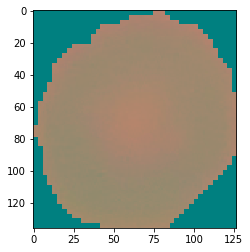In [ ]: